# The Retired Professor Simplifies Algebra: Linear Equations

## Algebra simplified into a brief but thorough free resource can benefit both a parent helping a student and the student. This segment addresses linear equations.

The Retired Professor Simplifies is a series of topics designed to be brief presentations that will enable parents to assist in the learning process. The articles can be used directly by the student, or by a parent trying to explain an unfamiliar topic.

This is the first segment of a series that addresses algebra in a simple manner. The first part of the algebra series is linear equations, and it includes absolute value equations and special cases.

The parts of the series will have links to the other parts. Viewing other parts is sometimes necessary, since algebra builds on itself.

### Math Anxiety

Before we start it is important to address whether there is a problem with math anxiety.  If there is a belief by the student that it is impossible to learn math, that impediment must be addressed first.  Fortunately, there are some excellent books written on the subject that can really help.

### Linear Equations

Any equation has two expressions that are equal.  So, whatever is done to one side of the equation, if also done to the other, does not change the fact that the two expressions are equal.

The objective is to preform operations so as to cause the variable to be alone on one side of the equation, and not appear on the other side.

We will build complexity slowly.  In the end you will have seen different examples that will help with your understanding.

Solve for xx + 2 = 9

In order to get the variable alone on one side of the equation subtract 2 from each side.

x + 2 – 2 = 9 – 2, which gives x = 7.

Solve for x: 3x = 9

In order to get the variable alone on one side of the equation divide both sides by 3.

3x/3 = 9/3, which gives x = 3.

Solve for x: 2x + 1 = 4x - 7

Think that x stands for a number, so it can be treated as you would treat a number.

You must decide which way you want to send the variable, and send the number terms the other way.

2x – 2x + 1 + 7 = 4x – 2x – 7 + 7

8 = 2x

Now divide each side by 2.

8/2 = 2x/2, which gives 4 = x.

Note:  It really does not matter which side of the equation the variable ends up on.

Solve for x:  2(x + 3) = -x – 9

Always remove the parentheses, or other symbol of grouping, first.  This requires the distributive property, a(b + c) = ab + ac.

Eliminating the parentheses gives:  2x + 6 = -x – 9

Continue to solve.  2x + x + 6 + 9 = -x + x – 9 + 9

3x = 15

Divide by 3:  3x/3 = 15/3, which solves as x = 5.

Sometimes the variable disappears.  If the result is a true statement all values for the variable are solutions, or all real numbers solve the equation.  If the result is a false statement, the equation has no solution.

Solve for x:  2x + 5 = 3x + 5 – x

Collect like terms on each side of the equation.

2x + 5 = 2x + 5

Subtract 2x from each side of the equation.

2x – 2x + 5 = 2x – 2x + 5, or 5 = 5 (TRUE)  So, all real numbers are solutions.

Solve for x:  2x + 5 = 3x + 6 – x

Collect like terms on each side of the equation.

2x + 5 = 2x + 6

Subtract 2x from each side of the equation.

2x – 2x + 5 = 2x – 2x + 6, or 5 = 6 (FALSE)  So, the equation has no solution.

### Absolute Value

If we think about an absolute value, say │7│ or │-7│, we get the non-negative number the size of what is between the absolute value bars.  In this case either expression is equal to 7.

Now, we must allow a variable in the expression we wish to take the absolute value of.

Many textbooks solve with the two signs placed on the other side of the equation.

x│ = 6 is written x = 6 and x = -6.  While it may seem like a wasted extra step at this point, soon the reason for changing this to -x = 6 and x = 6.  The first equation can be easily solved for x as x = -6.

As an example, solve │2x + 5│ = 3.

There will be two equations, both linear equations.

-(2x + 5) = 3 and 2x + 5 = 3

First equation:  -(2x + 5) = 3

Multiply each side of the equation by -1.

(-1)( -(2x + 5)) = (-1)3

2x + 5 = -3

2x + 5 - 5 = -3 – 5

2x = -8

2x/2 = -8/2

x = -4

Second equation:

2x + 5 = 3

2x + 5 - 5 = 3 – 5

2x = -2

2x/2 = -2/2

x = -1

There are two solutions, -1 and -4.

Since an absolute value can never be negative, we can immediately write “no solution” for any absolute value that is equal to a negative value.

(In the above the negative values are for the variable, not the absolute value.)

As an example, suppose you are asked to solve the absolute value │x + 3│ = -4

Immediately write down “No solution exists.”  Solving the two equations is misleading, you will get solutions to those equations that will not solve the absolute value equation.

Now for the harder examples.

Solve │x + 3│ = │x + 4│

Here, we get four equations with all variations of positive and negative expressions.

x + 3 = x + 4, -(x + 3) = x + 4, x + 3 = -(x + 4), and –(x + 3) = -(x + 4)

If you multiply the third equation by -1 on each side it becomes the second equation.  Likewise, multiplying the fourth equation by -1 on both sides gives the first equation.  So, there are only two equations to consider.

x + 3 = x + 4

Subtracting x from both sides gives

x x + 3 = xx + 4

3 = 4, a false statement, which indicates this equation produces no solutions.

-(x + 3) = x + 4

Using the distributive law, we get

-x + (-3) = x + 4

-x + x + (-3) – 4 = x + x + 4 – 4

-7 = 2x

Dividing by 2 we get

-7/2 = 2x/2

-7/2 = x, which is a solution of the absolute value equation.

### Other Topics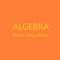This is the second part of a free resource to help with the basic understqanding of algebra. It can be used by students and those helping students witht the understanding.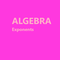This is a free resource on understanding algebra, and presented in a short presentation aimed at making things clear. It may help thoses studying algebra and those trying to help.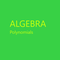This of a free resource where insights into algebra are provided to help both students and those helping students with algebra.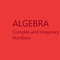This is a free to use algebra resource. It is designed to make it easy to understand complex and imaginary numbers for students and those assisting students with learning.

This article contains links to affiliate programs and Adsense advertising.  These must use cookies to allow for proper crediting, and allow me to earn from qualifying purchases. As an Amazon Associate I earn from qualifying purchases.

Updated: 02/09/2021, blackspanielgallery
1

Only logged-in users are allowed to comment. Login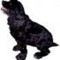blackspanielgallery on 02/08/2021

i probably will move on to trig or calculus in the future, but the pace will be slower. I had the algebra written for about a year, so it is easy to publish.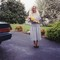DerdriuMarriner on 02/08/2021

blackspanielgallery, Thank you for the practicalities and products.
Your explanations for making sense of variables and for tackling false and true statements leading to no solutions and solutions are most helpful. It must be such a relief for children and parents, math-anxious or not, to access user-friendly books on numbers, not just on beginning math but on to algebra...and perhaps geometry, trigonometry and calculus?

#### You might also like

Learn Algebra and Trigonometry with Rap Videos

Learn the hard to grasp Algebra and Trigonometry basics from inspiring math t...

The Retired Professor Simplifies Algebra: Systems of Equations

Insights into algebra with attention to systems of linear equations is the fo...

Disclosure: This page generates income for authors based on affiliate relationships with our partners, including Amazon, Google and others.